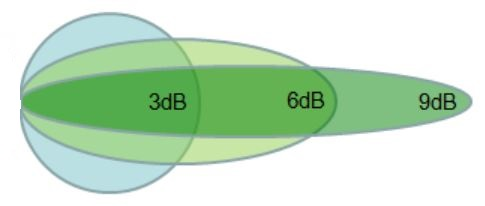dB, dBi, dBm dB(mW): Defined, Explained and Differentiated

dB refers to the decibel, which is the unit of measurement of sound though it is also a relative measure of the power between two levels. Therefore dB is not an absolute measurement but rather a ratio.

dB as a standalone unit represents loss or gain and does not have any dimension. It has to be referenced to something else to provide meaningful measurement.

So, the term "dB" is not a reference but is rather a method and a measurement standard. dB must be used against a standard:  dBi & dBm are dB (decibels) measured against a standard:

• dBi (decibels per isotropic dB[isotropic]) is a measure of the forward gain of an antenna: The gain in power emitted by an antenna signal.
• dBm (decibels per milliwatt): The relative power emitted by an amplifier:  Refers to decibels as a relative measure of milliwatts.
• There is no direct correlation between watts and dBi. dbm is logarithmic and mw is linear.

dBi  = dB(isotropic): The forward gain of an antenna compared with the hypothetical isotropic antenna, which uniformly distributes energy in all directions. Linear polarization of the EM field is assumed unless noted otherwise.

dBi refers to decibels measured against an isotropic reference antenna.  A good illustration of this is when you are using an application such as a WiFi router. In such an instance, dBi would refer to how effective the WiFi router’s antenna is. Therefore dBi is a term that is used when measuring the relative antenna gain of applications.

dBis tend to vary with the most common indoor WiFi router antennas designed to work at 4-9 dBi, while outdoor antennas range between 15 and 24 dBi. Outdoor antennas have better dBis than indoor antennas as they are directional antennas used in point to point and point to multipoint connections.

dBm dB(mW):  dBm is is an expression of power in decibels per milliwatt.  We use dBm when we are measuring power emitted from amplifiers. We measure that power in milliwatts which is typically abbreviated as mW.

As such, if you have a WiFi router, dBm measures the power the antenna is emitting, which can play a significant role in how much range the router has. Low dBms provide weak connections and may make working with such a router difficult or even impossible. On the other hand, very high dBms can result in the amplification of interference and background noise, which will result in a poor quality signal. Countries will typically have specific regulations on how much power an antenna may emit.

When used in audio work the milliwatt is referenced to a 600 ohm load, with the resultant voltage being 0.775 volts. When used in the 2-way radio field, the dB is referenced to a 50 ohm load, with the resultant voltage being 0.224 volts. There are times when spec sheets may show the voltage & power level e.g. -120 dBm = 0.224 microvolts.

dBm / dB(mW) is sometimes referred to as decibel milliwatts. The reference finds application in fiber optical communication, microwave and radio networks to express absolute power. It is preferred since it can express both very small and very large values in short form as compared to the BW, whose smallest unit is 1000 mW – one watt.

The unit of measurement is used when measuring absolute power given that it is an absolute unit referenced to the watt. Comparatively, the dB (decibel) is a used for quantifying rations between values such as noise to signal ratio, which makes it a dimensionless unit. The fixed reference value of the dBmW is what makes it an absolute measure.For more key parameters of antenna evaluation: See VSWR and Front to Back Ratio

Posted by